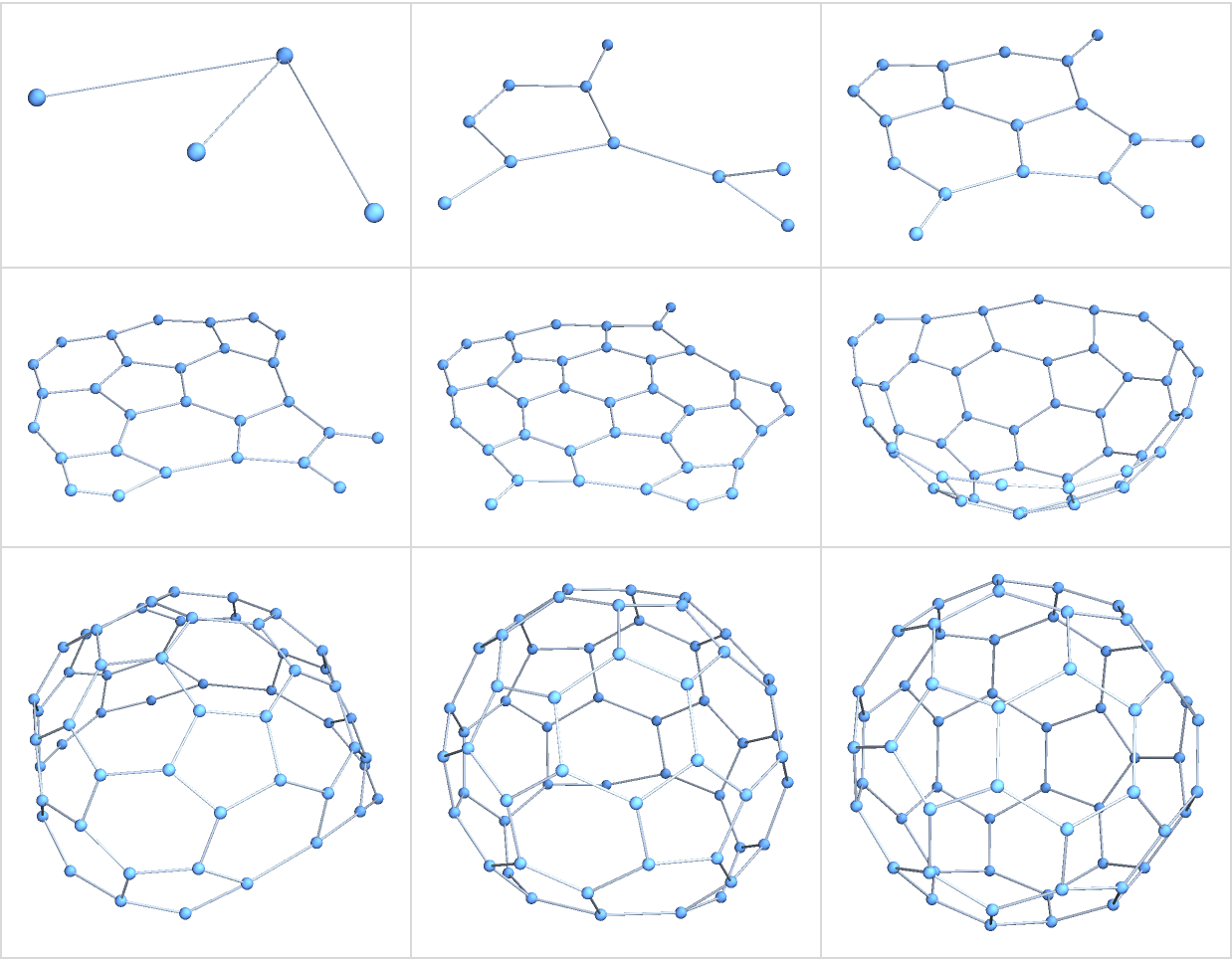This resource function is obsolete. Use the function NeighborhoodGraph instead.

Function Repository Resource:

# SubgraphExpand

Expand a subgraph inside the space of a graph

 ResourceFunction["SubgraphExpand"][g,{v1,v1,…},r] selects the subgraph of graph consisting of all edges between only those vertices of g whose GraphDistance through g to the nearest vertex vi is less than or equal to radius r. ResourceFunction["SubgraphExpand"][g,sg,r] accepts subgraph sg as the initial value (or kernel) of the expansion.

## Details

ResourceFunction["SubgraphExpand"] accepts the same options as Graph.

## Examples

### Basic Examples (2)

Expand a cycle graph around one vertex:

 In:=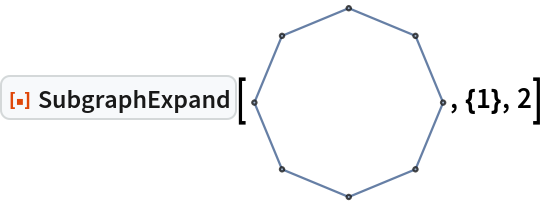Out=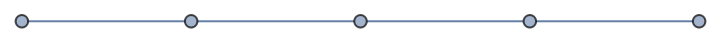Expand a grid graph around its central vertex at several radii:

 In:=Out=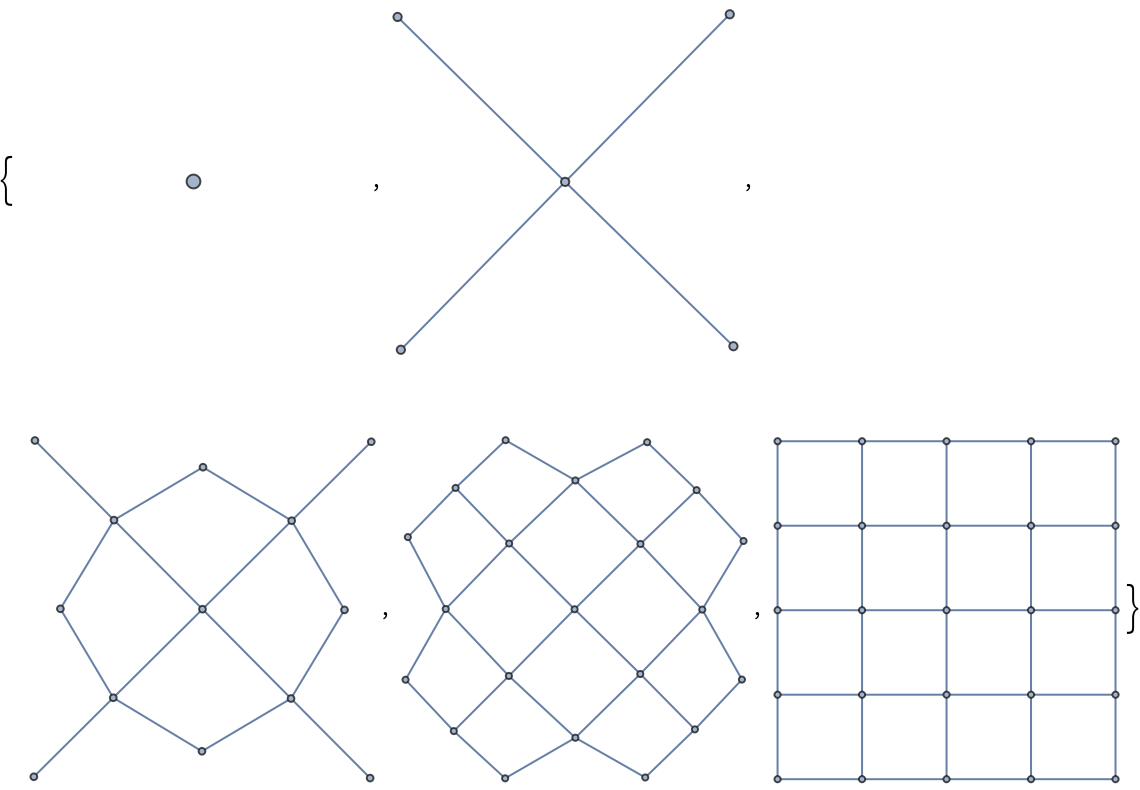Use directed edges to only expand the grid graph into the Northeast quadrant:

 In:=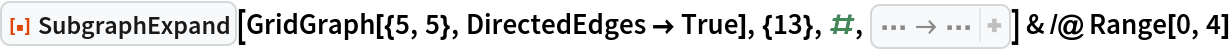Out=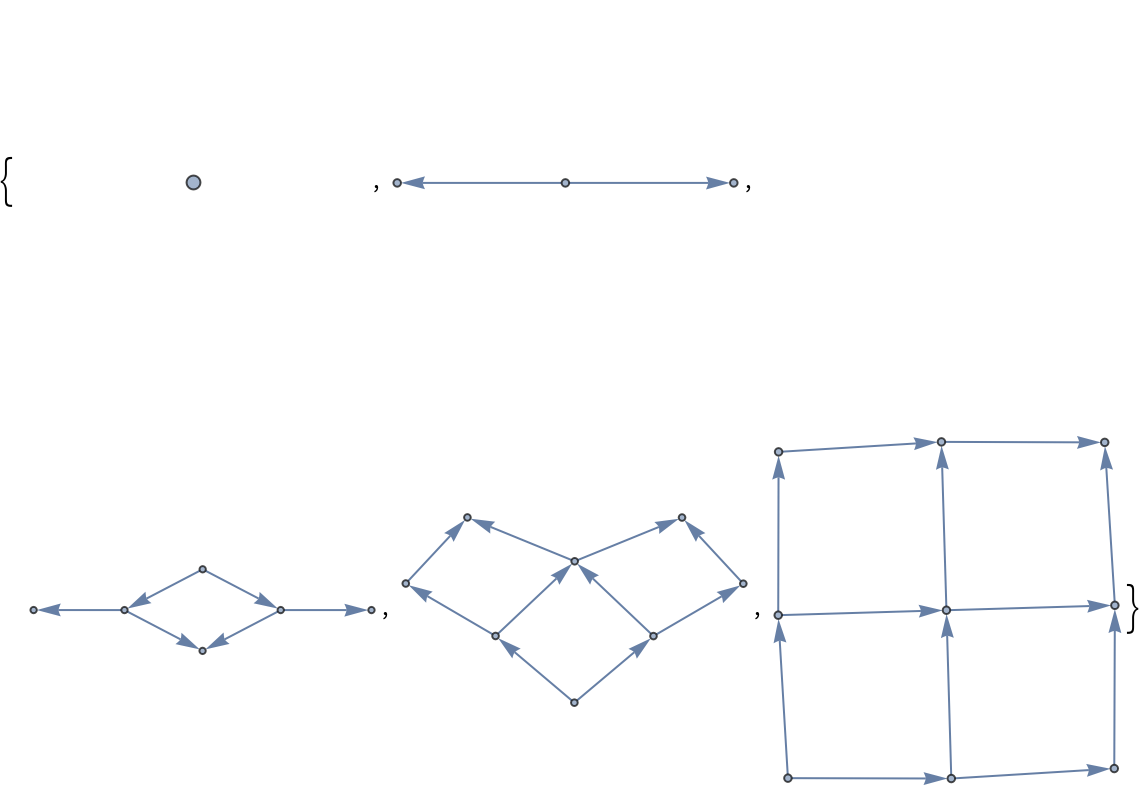### Scope (2)

Expand a graph around multiple initial vertices:

 In:=Out=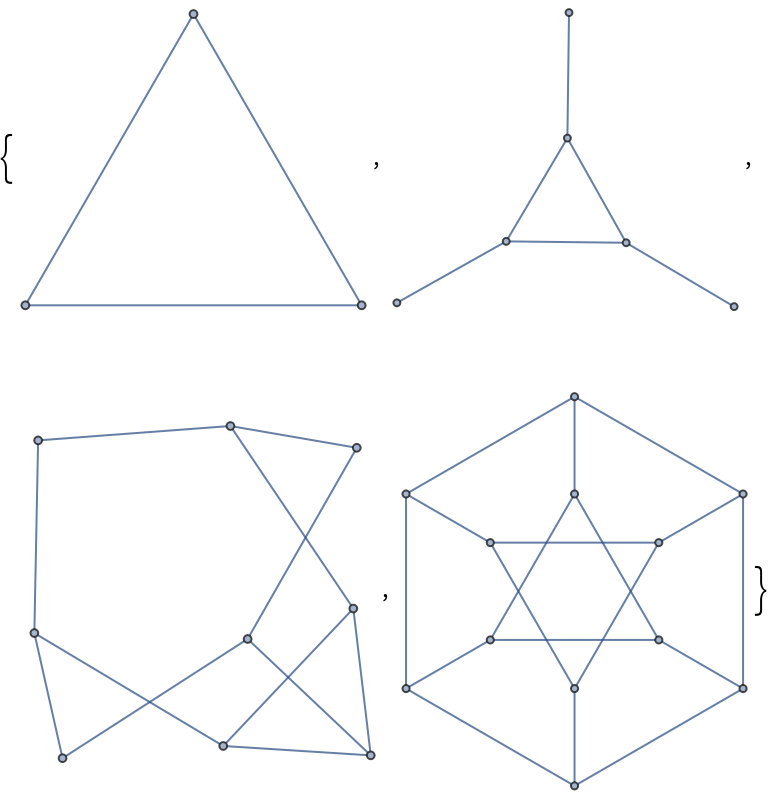Define a graph:

 In:=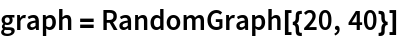Out=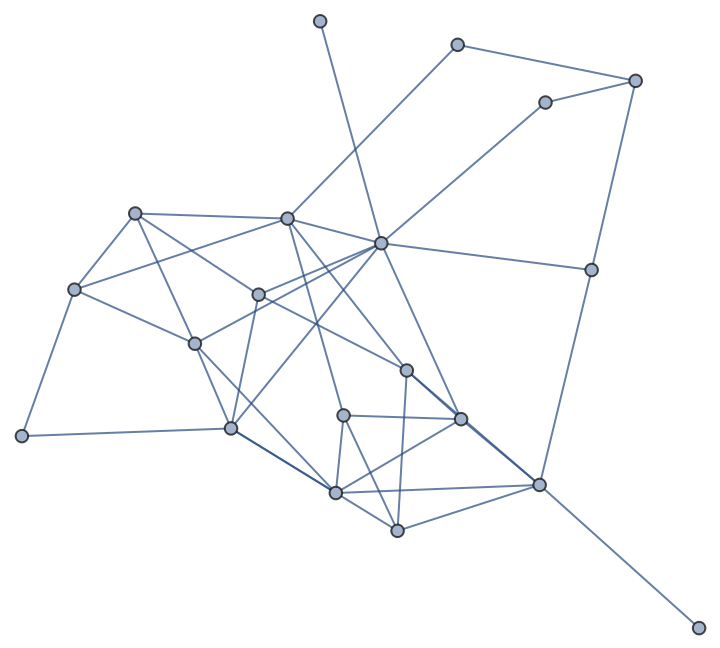Extract a subgraph:

 In:=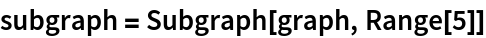Out=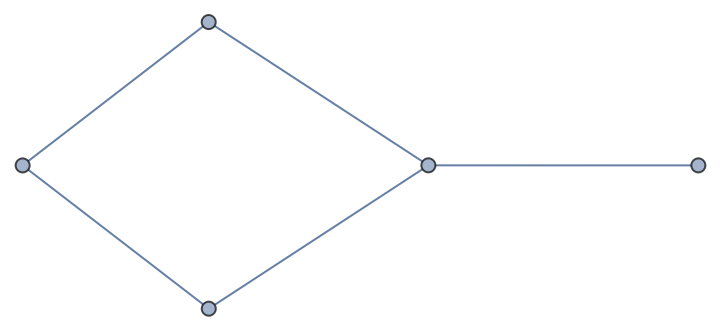Expand that subgraph by radius one:

 In:=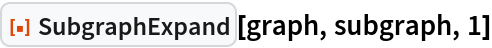Out=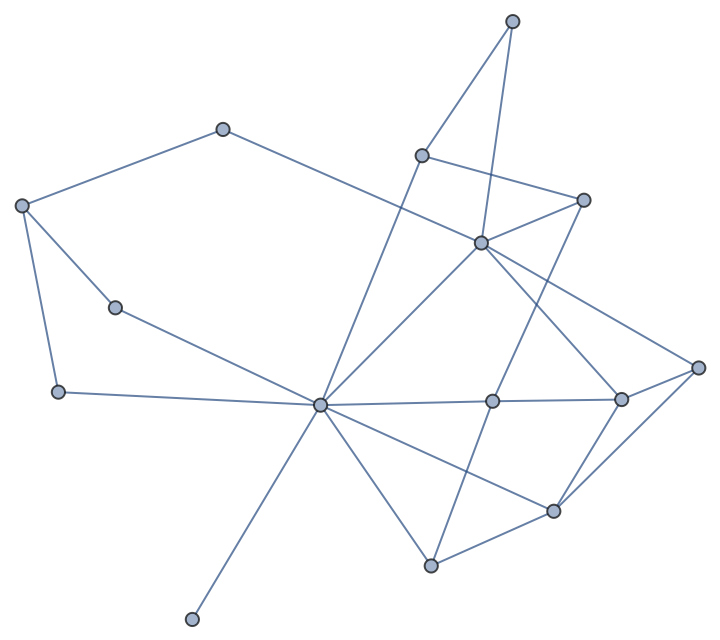### Possible Issues (2)

SubgraphExpand does not recognize subgraph isomorphism:

 In:=Out=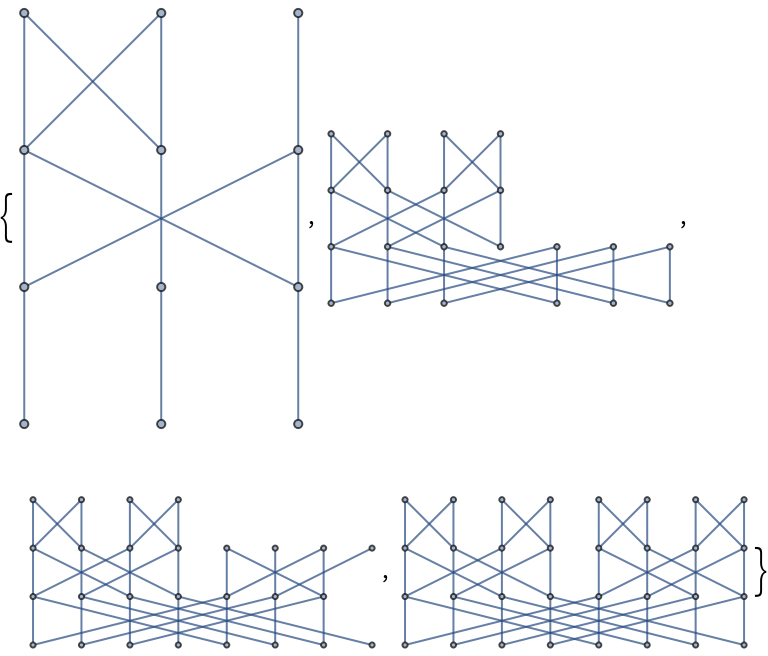This can be fixed by manually choosing vertices:

 In:=Out=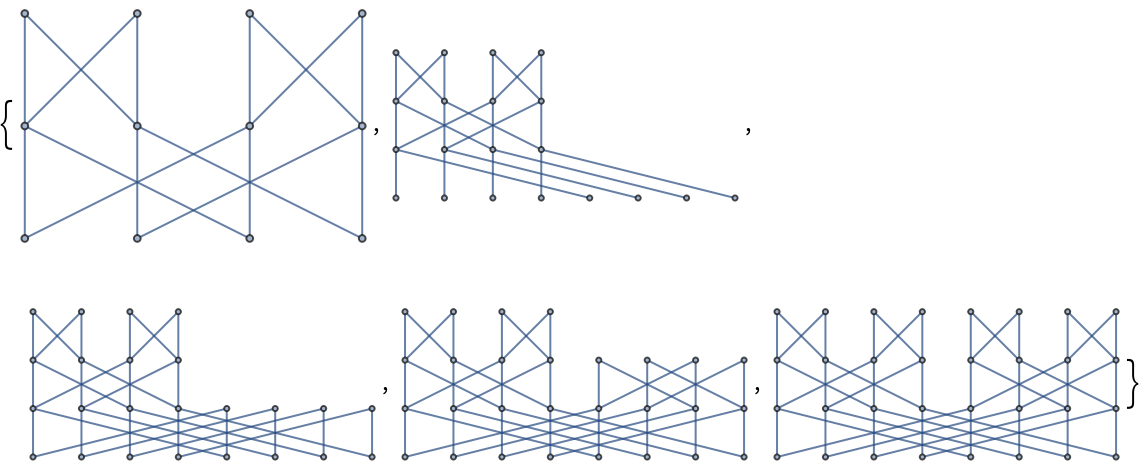### Neat Examples (1)

Expand a polyhedral skeleton graph into three dimensions:

 In:=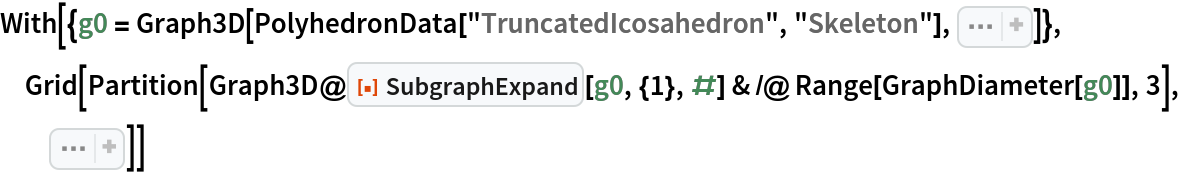Out=